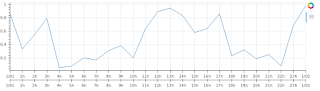I have a timeseries which times are in UTC, that I can show like that:

``````from bokeh.plotting import show, figure, output_notebook
from bokeh.models import ColumnDataSource as CDS, LinearAxis, Range1d,DatetimeTickFormatter
import pandas as pd
import pytz
import numpy as np
from datetime import timedelta
output_notebook()

index=pd.date_range('2018-1-1','2018-1-2',freq='H',tz='UTC')
data=np.random.rand(len(index))
df=pd.DataFrame(data,index,columns=['data']).reset_index()

source=CDS(df.to_dict('list'))
f=figure(width=900,height=250,x_axis_type='datetime')
f.xaxis.ticker.desired_num_ticks=24
f.line('index','data',source=source)

show(f)
``````

``

However, I would like to be able to add an additional x axis showing the datetime in a different timezone.

I tried:

``````tz = pytz.timezone('US/Eastern')
f.extra_x_ranges = {"orig_tz": Range1d(
start=source.data['index'].astimezone(tz),
end=source.data['index'].astimezone(tz)+timedelta(days=1) )}
f.xaxis.ticker.desired_num_ticks=24

show(f)
``````

``

But this displays:We can see that the second x axis starts at 1/1/2018 00:00:00 when its should start with the offset of 6 hours at 31/12/2017 18:00:00. It similarly ends on the wrong time (1/1/2018 00:00:00 instead of 1/1/2018 18:00:00)

I tried to check / change the `start` and `end` values in`f.extra_x_ranges['orig_tz']['property_values]` but i see they are correctly set.

How can I correctly display the second axis with (here in that example) a shift of 6 hours ?

Hi Vincent,

The default range is DataRange1d - it adds a small margin by default, that’s why you see some space around your line on the plot. You specify an extra range with explicit bounds - there’re no margins added in this case.

Also, when you specify axis type as “datetime”, the whole axis class is replaced with “DatetimeAxis” - you should use it for the extra axis as well so that both ticker and formatter are datetime-aware.

Here’s a fixed version of your code. Note also some minor changes regarding passing the data into a data source and regarding using data bounds instead of using timedelta.

import numpy as np
import pandas as pd
import pytz

from bokeh.models import ColumnDataSource, Range1d, DatetimeAxis
from bokeh.plotting import show, figure, output_file

index = pd.date_range(‘2018-1-1’, ‘2018-1-2’, freq=‘H’, tz=‘UTC’)
data = np.random.rand(len(index))
df = pd.DataFrame(data, index, columns=[‘data’])

source = ColumnDataSource(df)
f = figure(width=900, height=250, x_axis_type=‘datetime’, tools=‘hover’,
x_range=Range1d(start=df.index, end=df.index[-1]))
f.xaxis.ticker.desired_num_ticks = 24
f.line(‘index’, ‘data’, source=source)

tz = pytz.timezone(‘US/Eastern’)
f.extra_x_ranges = {“orig_tz”: Range1d(start=df.index.astimezone(tz),
end=df.index[-1].astimezone(tz))}
f.xaxis.ticker.desired_num_ticks = 24

``

Regards,

Eugene

···

On Monday, January 29, 2018 at 12:26:25 AM UTC+7, Vincent Konaté wrote:

I have a timeseries which times are in UTC, that I can show like that:

``````from bokeh.plotting import show, figure, output_notebook
from bokeh.models import ColumnDataSource as CDS, LinearAxis, Range1d,DatetimeTickFormatter
import pandas as pd
import pytz
import numpy as np
from datetime import timedelta
output_notebook()

index=pd.date_range('2018-1-1','2018-1-2',freq='H',tz='UTC')
data=np.random.rand(len(index))
df=pd.DataFrame(data,index,columns=['data']).reset_index()

source=CDS(df.to_dict('list'))
f=figure(width=900,height=250,x_axis_type='datetime')
f.xaxis.ticker.desired_num_ticks=24
f.line('index','data',source=source)

show(f)
``````

``

However, I would like to be able to add an additional x axis showing the datetime in a different timezone.

I tried:

``````tz = pytz.timezone('US/Eastern')
f.extra_x_ranges = {"orig_tz": Range1d(
start=source.data['index'].astimezone(tz),
end=source.data['index'].astimezone(tz)+timedelta(days=1) )}
f.xaxis.ticker.desired_num_ticks=24

show(f)
``````

``

But this displays:We can see that the second x axis starts at 1/1/2018 00:00:00 when its should start with the offset of 6 hours at 31/12/2017 18:00:00. It similarly ends on the wrong time (1/1/2018 00:00:00 instead of 1/1/2018 18:00:00)

I tried to check / change the `start` and `end` values in`f.extra_x_ranges['orig_tz']['property_values]` but i see they are correctly set.

How can I correctly display the second axis with (here in that example) a shift of 6 hours ?

Hi Eugene,

Thx a lot for your reply - sorry to raply so late, somehow the alert for your post didnt show up in my emails.

I tried your code, and the result is as follow:So as you can see, the 2 axis show up correctly, but the second axis is the same as the first (i.e. the hour should be shifted as per the timezone difference).

Would you have a solution for the second axis to show the correct shift in time?

Thanks again,

Vincent